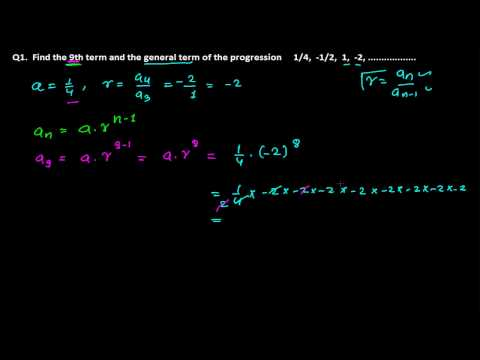## Geometric Sequences Problems – Based on Nth term -General Termitsmyacademy.com for free systematic study on geometric sequences. Nth term formula – general term formula we learnt in last video now we need to solve a very simple problem based on nth term or general term of the geometric sequences. It is quite easy we have to just put the value of a , r and the term number – n that we need to find in the given geometric sequence. where, a = first term of geometric sequence r = common ratio of geometric sequence n = term number of the geometric sequence we need to find I hope this tutorial be comes successful to give you a little idea of solving geometric sequences problem based on general term – nth term of geometric sequences.